Support

Apps

•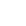My Wallet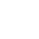My Order
•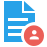My Profile
•My Connections
•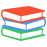My Books
•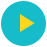My Videos
•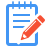My Tests
•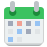My Calender
•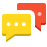My Messages
•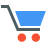My Shopping Cart
•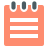My Orders
•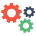Account Settings
•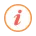Help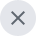# Verify Account

## A Verification Code has been sent to you registered email address shown below. Please enter the code to verify your account. You may edit the email address, if it is incorrect

Resend Code

Note: Please check your Spam or Junk folder, in case you didn't receive the email with verification code.

# Book Details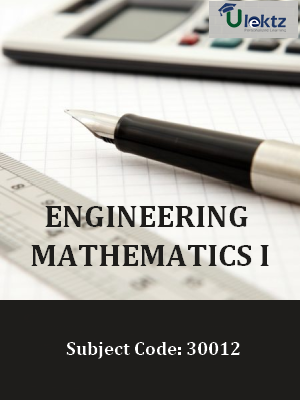# Engineering Mathematics-I

Published by uLektz

 Course Code : 30012 Author : uLektz University : Directorate of Technical Education,Tamilnadu Regulation : 2015 Categories : Engineering Mathematics Format :ePUB3 (DRM Protected) Type : eBook

FREE

Description :Engineering Mathematics-I of 30012 covers the latest syllabus prescribed by Directorate of Technical Education,Tamilnadu for regulation 2015. Author: uLektz, Published by uLektz Learning Solutions Private Limited.

Note : No printed book. Only ebook. Access eBook using uLektz apps for Android, iOS and Windows Desktop PC.

##### Topics
###### UNIT I ALGEBRA

1.1 DETERMINANTS: Definition and expansion of determinants of order 2 and 3.Properties of determinants (nor for examination). Solution of simultaneous equations using Cramer’s rule (in 2 and 3 unknowns)-Simple Problems

1.2 MATRICES: Definition - Singular Matrix, Non-singular Matrix, Ad joint of a matrix and inverse of a matrix up to 3 × 3 only. Simple problems. Definition – Rank of a matrix. Finding rank of a matrix by determinant method (matrix of order 3 × 4)

1.3 BINOMIAL THEOREM: Definition of Factorial notation - Definition of Permutation and Combinations - values of nPr and nCr (results only) (not for examination). Binomial theorem for positive integral index (statement only) - Expansion - Finding of general term, coefficient of xn and term independent of x. Simple Problems. Binomial Theorem for rational index up to - 3 (statement only). Expansion only for - 1, - 2 and - 3.

###### UNIT II COMPLEX NUMBERS

2.1 ALGEBRA OF COMPLEX NUMBERS: Definition – Real and Imaginary parts, Conjugates, Modulus and amplitude form, Polar form of a complex number, multiplication and division of complex numbers (geometrical proof not needed) – Simple Problems. Argan Diagram – Collinear points, four points forming square, rectangle, rhombus and parallelogram only. Simple problems.

2.2 DE MOIVRE’S THEOREM Demoivre’s Theorem (Statement only) – related simple problems.

2.3 ROOTS OF COMPLEX NUMBERS Finding the nth roots of unity - solving equation of the form xn ± 1 = 0 where n ≤ 7. Simple problems.

###### UNIT III TRIGONOMETRY

3.1 Expansion of sin (A ± B), cos (A ± B) and tan (A ± B) (without proof). Problems using above expansions.

3.2 Trigonometrical ratios of multiple angles of 2A and 3A and sub-multiple angles. Simple problems.

3.3 Trigonometrical ratios of sum and product formulae. Simple problems.

###### UNIT IV INVERSE TRIGONOMETRIC RATIOS AND DIFFERENTIAL CALCULUS-I

4.1 INVERSE TRIGONOMETRIC FUNCTIONS Definition of inverse trigonometric ratios – Relation between inverse trigonometric ratios. Simple problems

4.2 LIMITS: Definition of Limits. Problems using the following results & Simple Problem.

4.3 DIFFERENTIATION Definition – Differentiation of xn, sin x, cos x, tan x, cosec x, sec x, cot x, log x, ex, u ± v, uv, uvw, u/v (v ≠ 0) (results only) Simple problems using the above results.

###### UNIT V DIFFERENTIAL CALCULUS-II

5.1 DIFFERENTIATION METHODS Differentiation of function functions (chain rule), Inverse Trigonometric functions and Implict functions. Simple Problems.

5.2 SUCCESSIVE DIFFERENTIATION Successive differentiation up to second order (parametric form not included). Definition of differential equation, order and degree, formation of differential equation. Simple problems.

5.3 PARTIAL DIFFERENTIATION Definition – Partial differentiation of two variables up to second order only. Simple Problems.